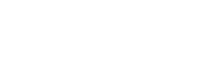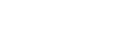## 使用KNN对数据进行分类

```import numpy as np
import pandas as pd
import matplotlib.pyplot as plt

print(df.shape)
X = df.drop('Outcome',axis=1).values
y = df['Outcome'].values

from sklearn.model_selection import train_test_split
X_train,X_test,y_train,y_test = train_test_split(X,y,test_size=0.4,random_state=42, stratify=y)

from sklearn.neighbors import KNeighborsClassifier

neighbors = np.arange(1,9)
train_accuracy =np.empty(len(neighbors))
test_accuracy = np.empty(len(neighbors))

for i,k in enumerate(neighbors):
#Setup a knn classifier with k neighbors
knn = KNeighborsClassifier(n_neighbors=k)

#Fit the model
knn.fit(X_train, y_train)

#Compute accuracy on the training set
train_accuracy[i] = knn.score(X_train, y_train)

#Compute accuracy on the test set
test_accuracy[i] = knn.score(X_test, y_test)

plt.title('k-NN Varying number of neighbors')
plt.plot(neighbors, test_accuracy, label='Testing Accuracy')
plt.plot(neighbors, train_accuracy, label='Training accuracy')
plt.legend()
plt.xlabel('Number of neighbors')
plt.ylabel('Accuracy')
plt.show()```## 分类指标评估

### Confusion Matrix 混淆矩阵```knn = KNeighborsClassifier(n_neighbors=7)
knn.fit(X_train,y_train)

from sklearn.metrics import confusion_matrix
y_pred = knn.predict(X_test)
confusion_matrix(y_test,y_pred)
pd.crosstab(y_test, y_pred, rownames=['True'], colnames=['Predicted'], margins=True)```

```Predicted    0   1  All
True
0          165  36  201
1           47  60  107
All        212  96  308```

### Classification Report

sklearn中的classification_report函数用于显示主要分类指标的文本报告。在报告中显示每个类的精确度，召回率，F1值等信息。

y_true：1维数组，或标签指示器数组/稀疏矩阵，目标值。
y_pred：1维数组，或标签指示器数组/稀疏矩阵，分类器返回的估计值。
labels：array，shape = [n_labels]，报表中包含的标签索引的可选列表。
target_names：字符串列表，与标签匹配的可选显示名称（相同顺序）。
sample_weight：类似于shape = [n_samples]的数组，可选项，样本权重。
digits：int，输出浮点值的位数。

```from sklearn.metrics import classification_report
print(classification_report(y_test,y_pred))

precision    recall  f1-score   support
0       0.78      0.82      0.80       201
1       0.62      0.56      0.59       107
avg / total       0.73      0.73      0.73       308```

#### 精确度＆召回率```from sklearn.metrics import precision_score
from sklearn.metrics import recall_score

print(precision_score(y_test, y_pred))
print(recall_score(y_test, y_pred))```

#### F1值### ROC曲线和AUC值

#### ROC

TP：正确肯定的数目
FN：漏报，没有正确找到的匹配的数目
FP：误报，给出的匹配是不正确的
TN：正确拒绝的非匹配对数FPR = FP/(FP + TN) 负样本中的错判率（假警报率）
TPR = TP/(TP + FN) 判对样本中的正样本率（命中率）
ACC = (TP + TN) / (P+N) 判对准确率ROC曲线和它相关的比率

P和N得分不作为特征间距离d的一个函数，随着阈值theta增加，TP和FP都增加。`In signal detection theory, a receiver operating characteristic (ROC), or simply ROC curve, is a graphical plot which illustrates the performance of a binary classifier system as its discrimination threshold is varied.````from sklearn.metrics import roc_curve

y_pred_proba = knn.predict_proba(X_test)[:,1]
fpr, tpr, thresholds = roc_curve(y_test, y_pred_proba)
plt.plot([0,1],[0,1],'k--')
plt.plot(fpr,tpr, label='Knn')
plt.xlabel('fpr')
plt.ylabel('tpr')
plt.title('Knn(n_neighbors=7) ROC curve')
plt.show()```#### AUC

ROC曲线是根据与那条参照线进行比较来判断模型的好坏，但这只是一种直觉上的定性分析，如果我们需要精确一些，就要用到AUC，也就是ROC曲线下面积。其判定方法是AUC应该大于0.5。如图所示：```from sklearn.metrics import roc_auc_score
roc_auc_score(y_test,y_pred_proba)```

`0.7345050448691124`

### GridSearchCV 网格搜索

GridSearchCV ，它存在的意义就是自动调参，只要把参数输进去，就能给出最优化的结果和参数。但是这个方法适合于小数据集，一旦数据的量级上去了，很难得出结果。这个时候就是需要动脑筋了。数据量比较大的时候可以使用一个快速调优的方法——坐标下降。它其实是一种贪心算法：拿当前对模型影响最大的参数调优，直到最优化；再拿下一个影响最大的参数调优，如此下去，直到所有的参数调整完毕。这个方法的缺点就是可能会调到局部最优而不是全局最优，但是省时间省力，巨大的优势面前，还是试一试吧，后续可以再拿bagging再优化。回到sklearn里面的GridSearchCV，GridSearchCV用于系统地遍历多种参数组合，通过交叉验证确定最佳效果参数。

```from sklearn.model_selection import GridSearchCV

param_grid = {'n_neighbors':np.arange(1,50)}
knn = KNeighborsClassifier()
knn_cv= GridSearchCV(knn,param_grid,cv=5)
knn_cv.fit(X,y)
print(knn_cv.best_score_)
print(knn_cv.best_params_)```

```0.7578125
{'n_neighbors': 14}```